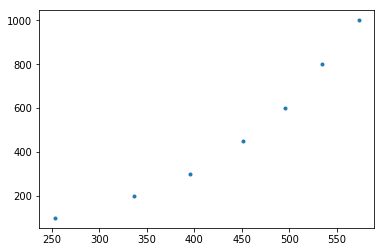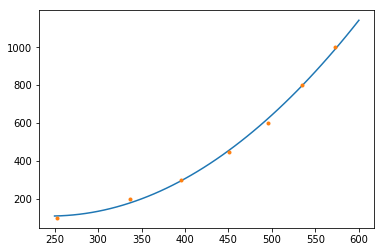# Modeling Galileo's ramp data¶

In :
%matplotlib inline
import matplotlib.pyplot as plt
import numpy as np
import pandas as pd
from scipy.linalg import solve


Beginning in 1595, Galileo began a series of experiments on projectile motion. In one such experiment, he let a ball roll down an inclined ramp right off of a table and measured it's vertical distance travelled. He did this for several ramp heights and thus obtained a relationship between the height at which he released the ball and the vertical distance that the ball traveled. I've got the actual data stored on my website! We can load and view it directly with Python like so:

In :
df = pd.read_csv('https://marksmath.org/data/galileos_ramp.csv')
df

Out:
H D
0 1000 573
1 800 534
2 600 495
3 450 451
4 300 395
5 200 337
6 100 253

The unit of distance is the "punti", according to the source:

We can also plot the data:

In :
plt.plot(df.D,df.H, '.')

Out:
[<matplotlib.lines.Line2D at 0x61932aef0>]While it rather flips the role of what we might call explanatory and response, it does look like height released is related to distance traveled via a quadratic - that is, $$H = a D^2 + b D + c.$$ In order to fit the data, we could solve the least squares problem $$\left(\begin{matrix} d_1^2 & d_1 & 1 \\ d_2^2 & d_2 & 1 \\ \vdots & \vdots & \vdots \\ d_n^2 & d_n & 1 \end{matrix}\right) \left(\begin{matrix}a \\ b \\ c \end{matrix} \right) \approx \left(\begin{matrix} h_1 \\ h_2 \\ \vdots \\ h_n \end{matrix}\right).$$

Of course, using the normal equations $A^T A \vec{x} = A^T \vec{b}$, this boils down to solving the linear system

$$\left(\begin{matrix} d_1^2 & d_2^2 & \cdots & d_n^2 \\ d_1 & d_2 & \cdots & d_n \\ 1 & 1 & \cdots & 1 \end{matrix}\right) \left(\begin{matrix} d_1^2 & d_1 & 1 \\ d_2^2 & d_2 & 1 \\ \vdots & \vdots & \vdots \\ d_n^2 & d_n & 1 \end{matrix}\right) \left(\begin{matrix}a \\ b \\ c \end{matrix} \right) = \left(\begin{matrix} d_1^2 & d_2^2 & \cdots & d_n^2 \\ d_1 & d_2 & \cdots & d_n \\ 1 & 1 & \cdots & 1 \end{matrix}\right) \left(\begin{matrix} h_1 \\ h_2 \\ \vdots \\ h_n \end{matrix}\right).$$

Here's how to set up the matrix containing the values of $D$ - the matrix traditionally called $A$ in the normal equations:

In :
A = np.array([df.D**2, df.D, df.D**0]).transpose()
A

Out:
array([[328329,    573,      1],
[285156,    534,      1],
[245025,    495,      1],
[203401,    451,      1],
[156025,    395,      1],
[113569,    337,      1],
[ 64009,    253,      1]])

We now solve the normal equations:

In :
a,b,c = solve(A.transpose().dot(A), A.transpose().dot(df.H))
a,b,c

Out:
(0.008180739771574061, -4.004842973935703, 600.0537247468947)

Thus we expect $$H \approx 0.00818073977 D^2 - 4.00484297 D + 600.05.$$ Let's define and plot that function together with data:

In :
def f(x): return a*x**2 + b*x + c
xs = np.linspace(250,600)
ys = f(xs)
plt.plot(xs,ys)
plt.plot(df.D,df.H, '.')

Out:
[<matplotlib.lines.Line2D at 0x61ab5fe80>]Looks pretty good!